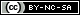# Weibull++ Examples

(Difference between revisions)
 Revision as of 06:55, 15 August 2012 (view source)← Older edit Revision as of 00:54, 16 August 2012 (view source) (→Life Data Analysis)Newer edit → Line 9: Line 9: ==Life Data Analysis== ==Life Data Analysis== *Simple Probability Plotting Examples: [[Probability Plotting Example|Simple Example]], [[3-Parameter_Weibull_Example|3-P Weibull Example]], [[1P_Exponential_Example|1-P Exponential]], [[Normal Distribution Probability Plotting Example|Normal]], [[Example:_Lognormal_Distribution_Probability_Plot|Lognormal]] *Simple Probability Plotting Examples: [[Probability Plotting Example|Simple Example]], [[3-Parameter_Weibull_Example|3-P Weibull Example]], [[1P_Exponential_Example|1-P Exponential]], [[Normal Distribution Probability Plotting Example|Normal]], [[Example:_Lognormal_Distribution_Probability_Plot|Lognormal]] + *[[Standard_Folio_Plots|Standard Folio Plots]] *Examples by Distribution: *Examples by Distribution: ::*[[Weibull Distribution Examples|Weibull]] ::*[[Weibull Distribution Examples|Weibull]] Line 23: Line 24: ::* Two Failure Modes Example. View it in '''[http://www.reliasoft.com/Weibull/examples/rc10/index.htm HTML]''' or        '''[http://www.reliasoft.tv/weibull/appexamples/weibull_app_ex_10.html Video]''' ::* Two Failure Modes Example. View it in '''[http://www.reliasoft.com/Weibull/examples/rc10/index.htm HTML]''' or        '''[http://www.reliasoft.tv/weibull/appexamples/weibull_app_ex_10.html Video]''' ::* [[Complex_Failure_Modes_Example|Complex Failure Modes Example]] ::* [[Complex_Failure_Modes_Example|Complex Failure Modes Example]] - *[[Standard_Folio_Plots|Standard Folio Plots]] ==Degradation Data Analysis== ==Degradation Data Analysis==

## Life Data Analysis

• Crack Propagation Example (Point Estimation)
View it in HTML or Video

## Recurrent Event Data Analysis

View it in HTML or Video

## Warranty Data Analysis

View it in HTML or Video

## UtilitiesContent on this site is available/licensed under the "Creative Commons Attribution-NonCommercial-ShareAlike 4.0 International License." See Copyright Information for details.